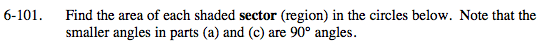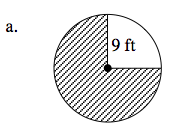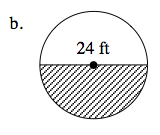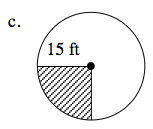### Home > MC2 > Chapter 6 > Lesson 6.2.3 > Problem6-101

6-101.A circle has a total measure of 360º. To find the area of the shaded sector, you must find the fraction of the circle that is shaded.

If the smaller angle of the non-shaded area is 90º, the shaded portion's angle must be 270º.

$\text{270\degree as a portion of 360\degree} = \frac{270\degree}{360\degree} = \frac{3}{4}$

This portion represents the fraction of the area that the shaded sector represents in the circle.

The area of a circle is π(r2).

Substituting in 9 ft for the radius, we get about 254.4 ft2 for the total area of the circle.

$\frac{3}{4} \text{ of 254.4 ft^2 = about 190.8 ft^2}$See part (a).176.6 ft2